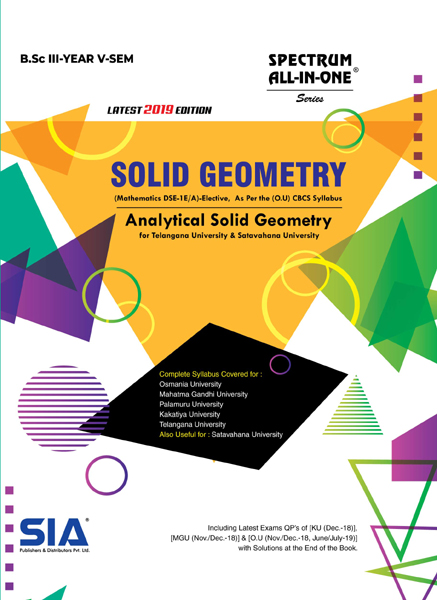•My WalletMy Order
•My Profile
•My Connections
•My Books
•My Videos
•My Tests
•My Calender
•My Messages
•My Shopping Cart
•My Orders
•Account Settings
•Help

# Book Details# Solid Geometry

 Course Code : B.Sc III-V (O.U) Author : SIA PUBLISHERS University : Osmania University Regulation : 2019 Categories : Arts and Science Format :PDF (DRM Protected) Type : eBook

Rs.132 Rs.39 Rs.71% off

Description :

SYLLABUS (O.U)

Unit-1

Sphere: Definition-The Sphere through four given points - Equations of a circle – Intersection of a sphere and a line-Equation of a tangent plane – Angle of intersection of two spheres – Radical plane.

Unit-2

Cones and Cylinders: Definition-Condition that the general equation of second degree represents a Cone-Cone and a plane through its vertex-Intersection of a line with a cone – The right circular cone – The cylinder – The right circular cylinder.

Unit-3

The Conicoid: The general equation of the second degree – Intersection of line with a Conicoid – Plane of contact – Enveloping cone and cylinder.

SYLLABUS (K.U)

Unit-1

Spheres: Definition-The Sphere through four given points - Equation of a circle – Intersection of a sphere and a line-Equation of a tangent plane – Angle of intersection of two spheres – Radical plane.

Unit-2

Cones: Definition-Condition that the general equation of second degree represents a Cone-Cone and a plane through

its vertex-Intersection of a line with a cone – The right circular cone.

Unit-3

Cylinders: Definition-Equation of a Cylinder-Enveloping cylinder – The Cylinder – The right circular cylinder.

Unit-4

The Conicoid: The general equation of the second degree – Intersection of line with a Conicoid – Plane of contact – Enveloping cone and cylinder.

Note : Solid Geometry, B.Sc III-V (O.U)

##### Publisher Detail:

Publisher Name: SIA Publishers and Distributors (P) Ltd.

Contact Email Id: msultan@siaedugroup.com